# 机器学习基础 ¶

1788 个字 预计阅读时间 6 分钟

Abstract

• 超算小学期第八次课课件

## 机器学习基础 ¶

### 线性回归 ¶

• 一维：$$\hat{y} = wx + b$$
• N 维：$$\hat{y} = \mathbf{w}^\top\mathbf{x} + b$$
• 损失函数：$$l^{(i)}(\mathbf{w}, b) = \dfrac{1}{2}(\hat{y}^{(i)}-y^{(i)})^2$$
• 梯度下降法求解参数

### 梯度下降 ¶

• $$L(\mathbf{w}, b) = \sum_il^{(i)}(\mathbf{w}, b)$$
• $$(\mathbf{w}, b)-\eta\nabla L(\mathbf{w}, b)$$ 代替 $$(\mathbf{w}, b)$$
• 学习率 $$\eta$$，超参数（用来控制学习），下降的快慢
• 学习率过大会在最优附近横跳
• 学习率过小则太慢
• 根据进度更改学习率
• Linear Decay
• Linear Warmup：先小，然后增大，再缓慢变小

### SGD¶

• 随机采用一部分样本来计算损失函数
• $$L(\mathbf{w}, b) = \sum_{i\in B}l^{(i)}(\mathbf{w}, b)$$
• Batch size $$s=|B|$$也是一个超参数
• $$s$$ 小，工作量小，拟合效果不好
• $$s$$ 大，内存消耗高

## 深度学习基础 ¶

### 多层感知器 ¶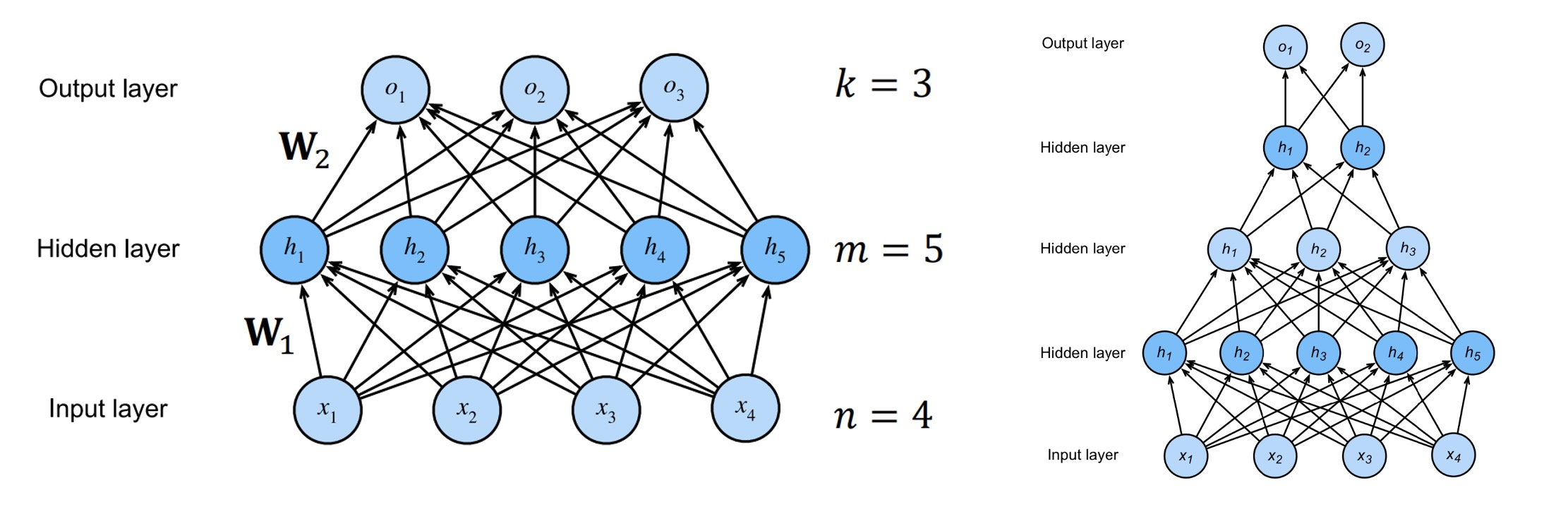• 输入层（Input Layer）：$$\mathbf{x}\in\mathbb{R}^n$$
• 隐藏层（Hidden Layer）：$$\mathbf{h} = \sigma(\mathbf{W_1x}+\mathbf{b_1})$$
• $$\mathbf{W_1}\in\mathbb{R}^{m\times n}, \mathbf{b_1}\in\mathbb{R}^m, \mathbf{h}\in\mathbb{R}^m$$
• $$\sigma$$：激活函数（activation function）
• 可以有很多隐藏层，逐层传递
• 输出层（Output Layer）：$$\mathbf{o} = \mathbf{W_2h}+\mathbf{b_2}$$
• $$\mathbf{W_2}\in\mathbb{R}^{k\times m}, \mathbf{b_2}\in\mathbb{R}^k, \mathbf{o}\in\mathbb{R}^k$$
• 结果：$$\mathbf{\hat{y}}=\sigma_2(\mathbf{o})$$

#### 反向传播（Back Propagation）¶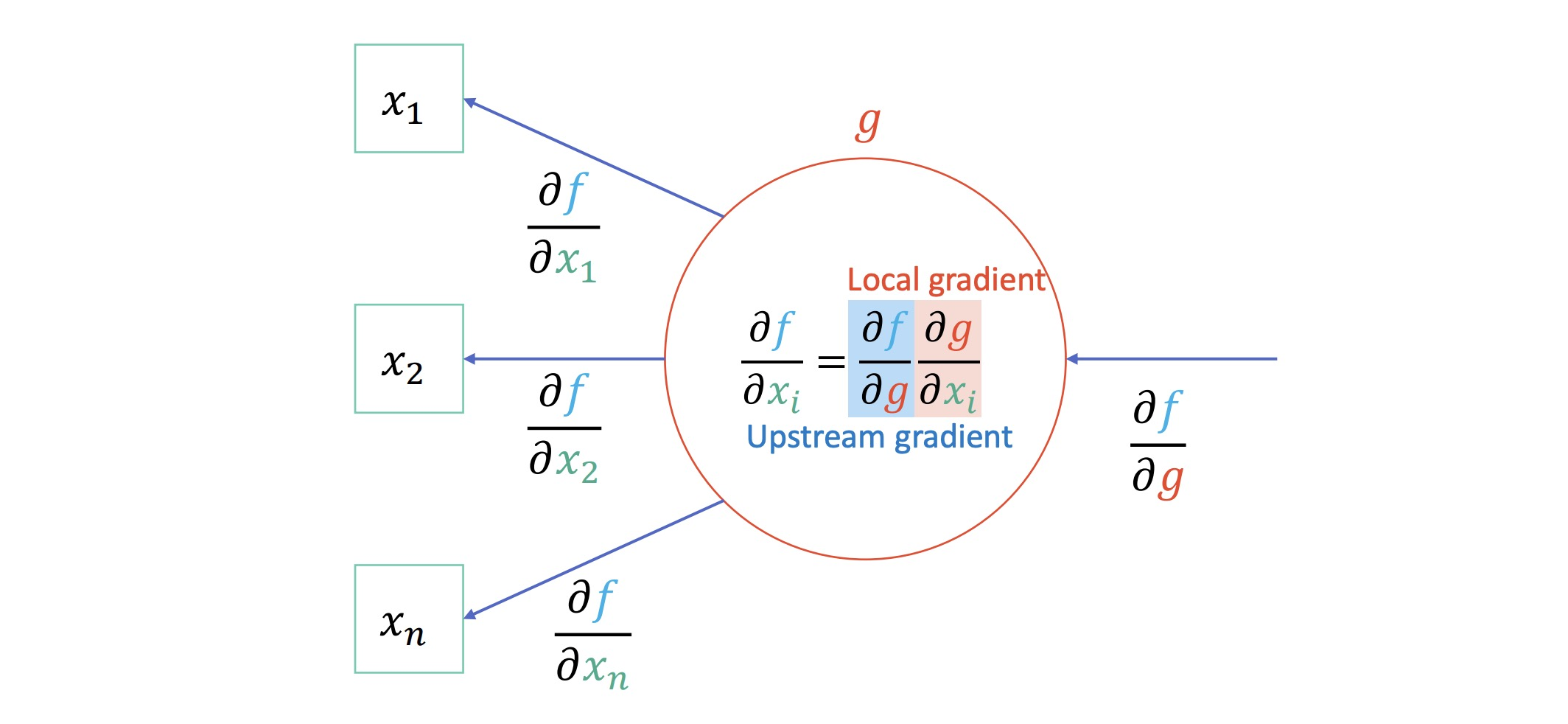### 激活函数 ¶

• $$\mathbf{h} = \mathbf{W_1x}+\mathbf{b_1}$$
• $$\mathbf{o} = \mathbf{W_2h}+\mathbf{b_2} = \mathbf{W_1W_2x}+\mathbf{b_1}+\mathbf{b_2}$$ 仍为线性的

• sigmoid$$\text{sigmoid}(x) = \dfrac{1}{1+e^{-x}}$$，导数：$$\text{sigmoid}(x)(1-\text{sigmoid}(x))$$
• tanh$$\tanh(x) = \dfrac{1-e^{-2x}}{1+e^{-2x}}$$，导数：$$1-\tanh^2(x)$$
• ReLU$$\text{ReLU}(x)=\max(x, 0)$$
• Rectified Linear Unit
• 屏蔽掉负值
• softmax$$\text{softmax}(\mathbf{o})_i = \dfrac{e^{o_i}}{\sum_{j=1}^ke^{o_j}}$$
• 将输出的值转化为概率（和为 1

### 规范化 ¶

#TODO，PPT 55-59 页，没懂

### 优化算法 ¶

SGD：$$W\leftarrow W-\eta\nabla L(W)$$

#### SGD + Momentum¶

SGD 只依赖当前 batch 计算得到的梯度来更新，不稳定。在梯度下降过程中加入动量，累积历次计算的梯度，使之更稳定，而且防止陷入局部最优解

• $$v\leftarrow \rho v+\nabla L(W)$$
• $$v$$："velocity"，累积的梯度
• $$\rho$$："friction"，动量因子，一般为 0.9 0.99
• $$W\leftarrow W-\eta v$$

• $$s\leftarrow s+(\nabla L(W))^2$$
• $$s$$：累积平方梯度
• 平方是逐元素相乘
• $$W\leftarrow W-\dfrac{\eta}{\sqrt{s}+\epsilon}\nabla L(W)$$
• $$\epsilon$$：为了维持数值稳定性，一般为 $$10^{-6}$$$$10^{-7}$$ 之类小常数

• 迭代次数记为 $$i=1, \dots, n$$
• $$v\leftarrow \beta_1v+(1-\beta_1)\nabla L(W)$$
• $$s\leftarrow \beta_2s+(1-\beta_2)(\nabla L(W))^2$$
• Bias correction：$$v'=\dfrac{v}{1-\beta_1^i}, s'=\dfrac{s}{1-\beta_2^i}$$
• $$W\leftarrow W-\eta\dfrac{v'}{\sqrt{s'}+\epsilon}$$
• 一般情况下通常设 $$\beta_1=0.9, \beta_2=0.999, \eta=10^{-3}$$$$5\times 10^{-4}$$

## 卷积神经网络 ¶

### 卷积 ¶

$(f*g)(x)=\int_{-\infty}^\infty f(\tau)g(t-\tau)\mathrm d\tau$

$(f*g)(n)=\sum_{m=-\infty}^\infty f(m)g(n-m)$

$(f*g)(i, j)=\sum_a\sum_bf(a, b)g(i-a, j-b)$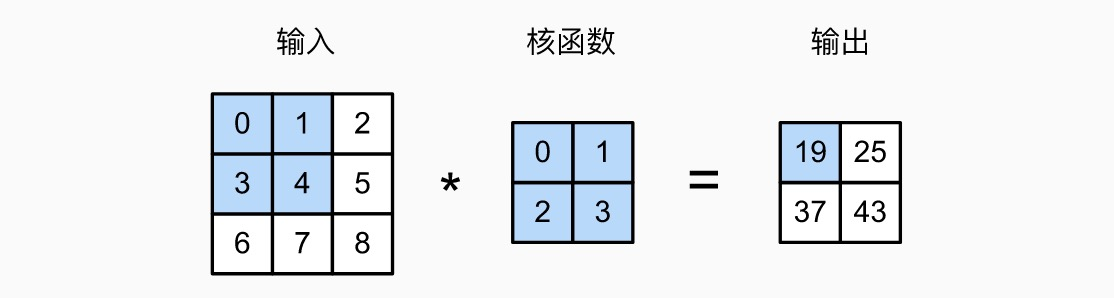• 在周围填充
• 可以填充 0，也可以填充临近的数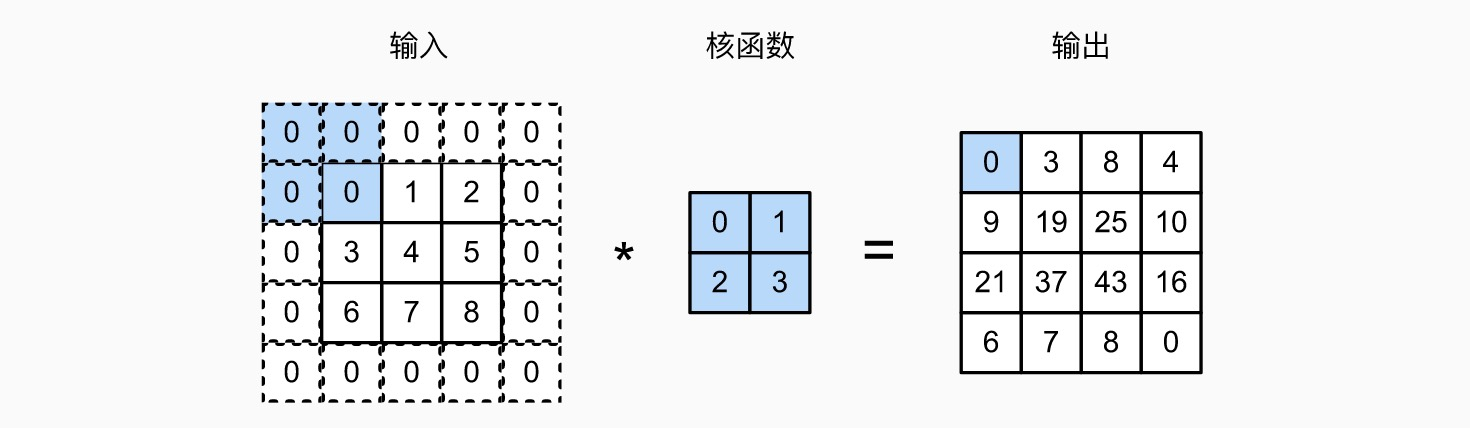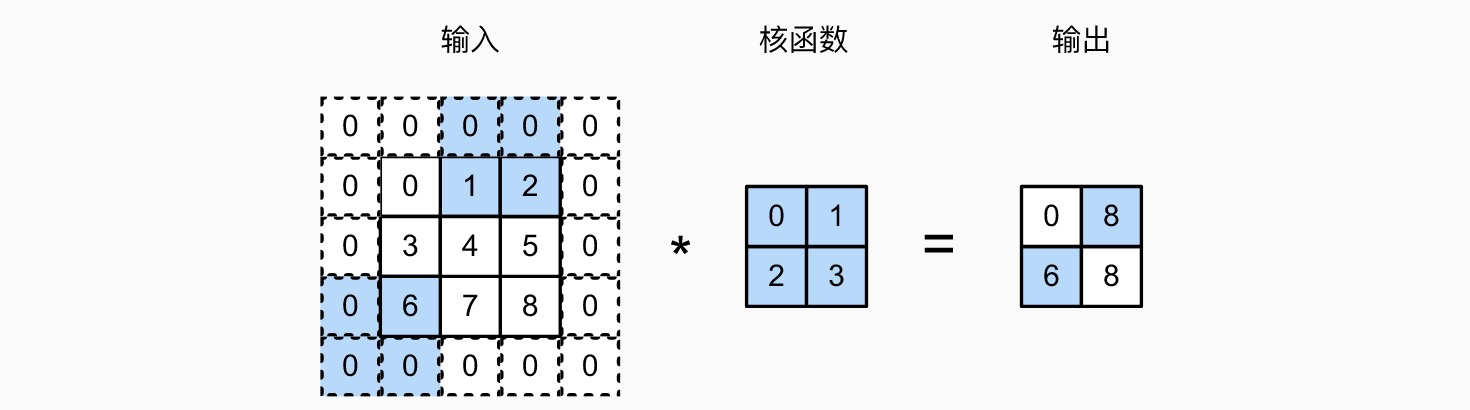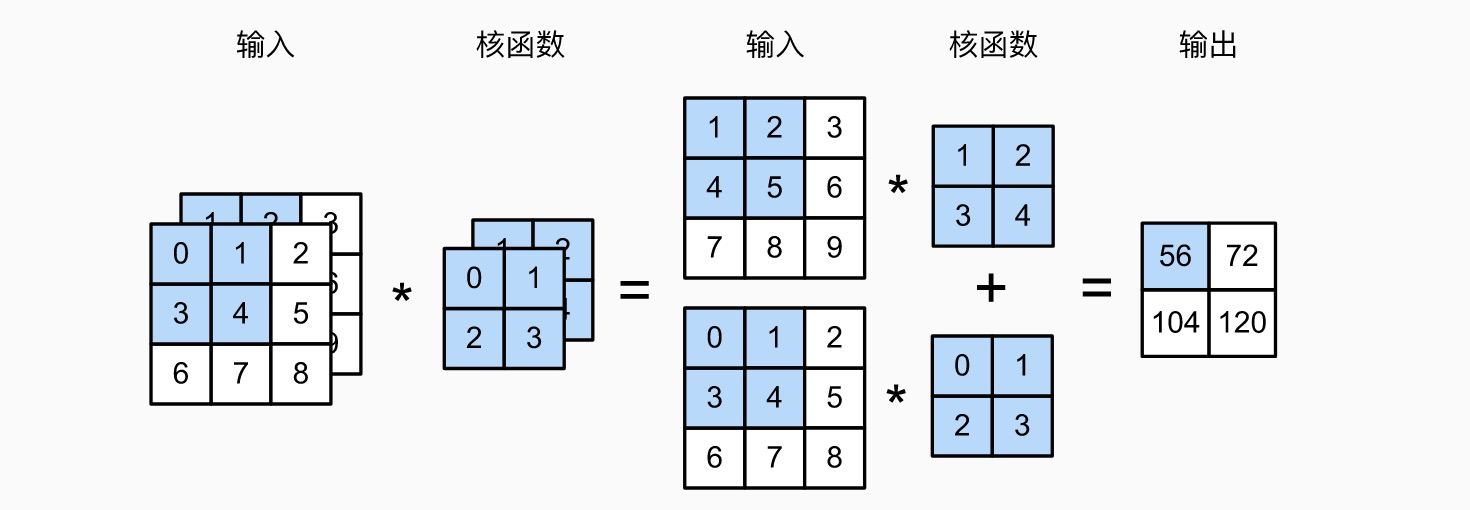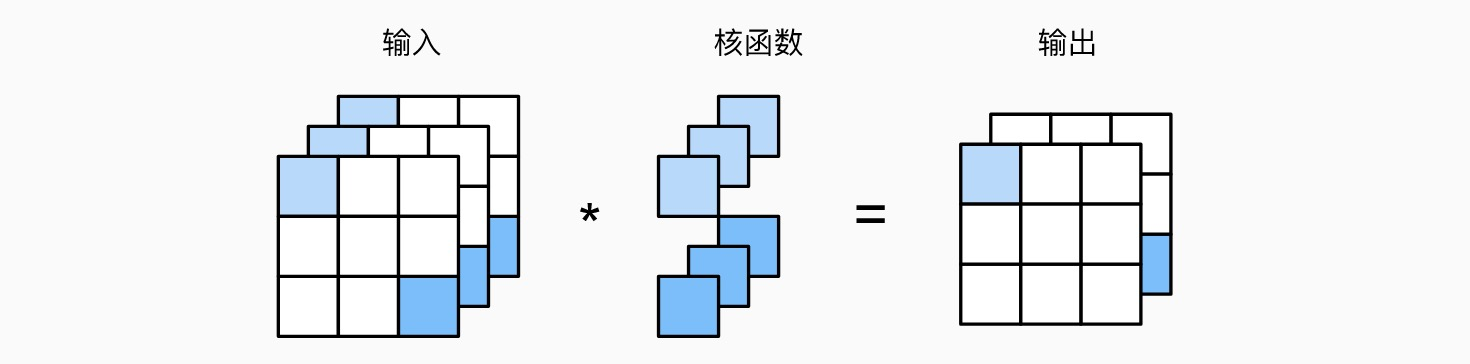### 汇聚（池化）¶### LeNet¶

1989 年提出的 CNN 模型（LeNet-5），用来从 28*28 的输入图片中识别数字，结构：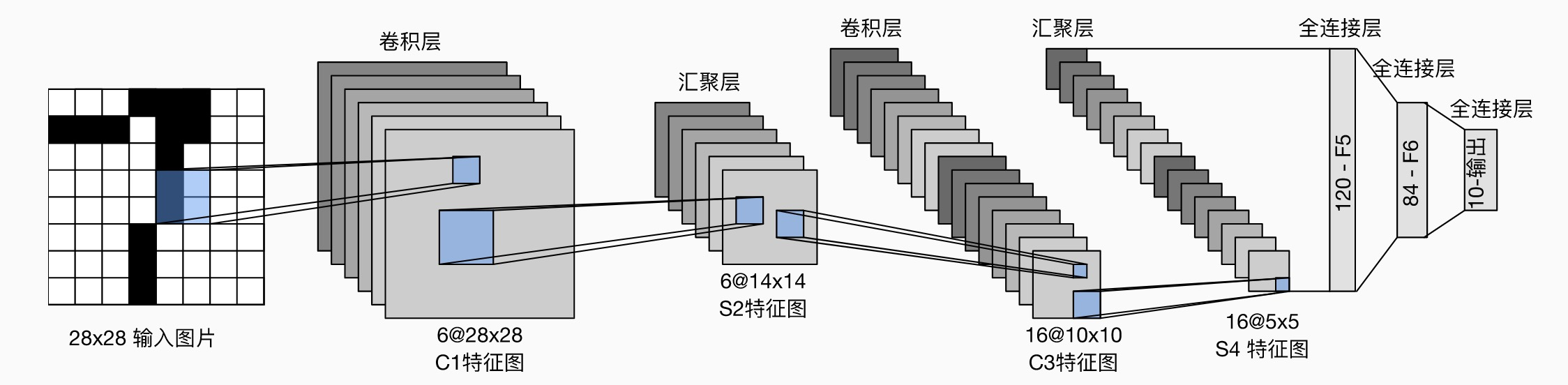• 卷积编码器：每个卷积块是一个卷积层（5*5 卷积核，padding 2），一个 sigmoid 激活函数，一个平均汇聚层（2*2 池，stride 2
• 第一层有 6 个通道，第二层有 16 个通道
• 全连接层密集块：有三个全连接层（中间是 sigmoid 激活函数）
• 三个全连接层输出个数依次为 120、84、10（最终 10 个数字）

### 现代卷积神经网络 ¶

#### AlexNet¶

LeNet 结构和设计理念类似。输入一个 224*224 带三通道的图片，输出识别出的物体类别（一共 1000 种），结构：

#### VGG¶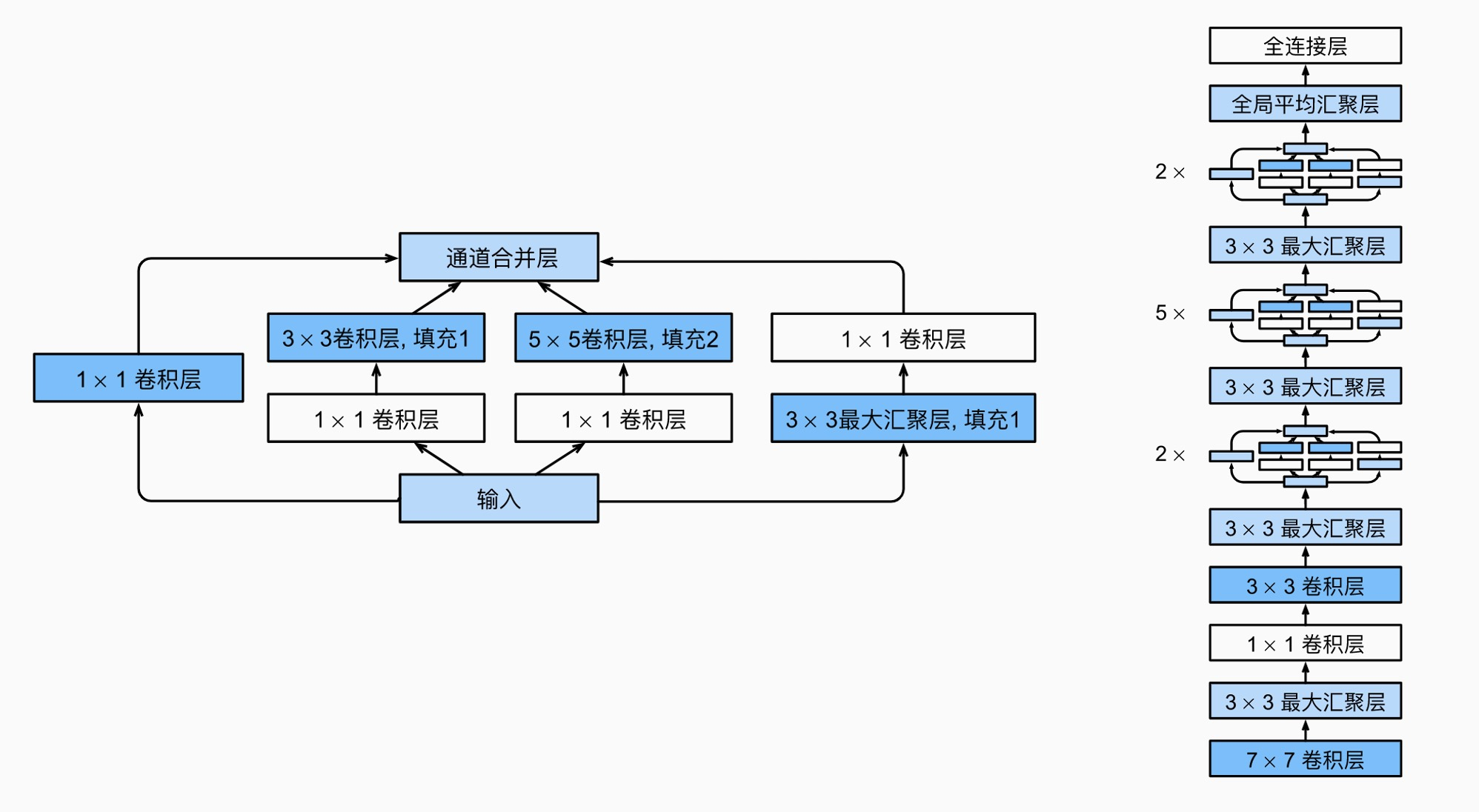#### ResNet¶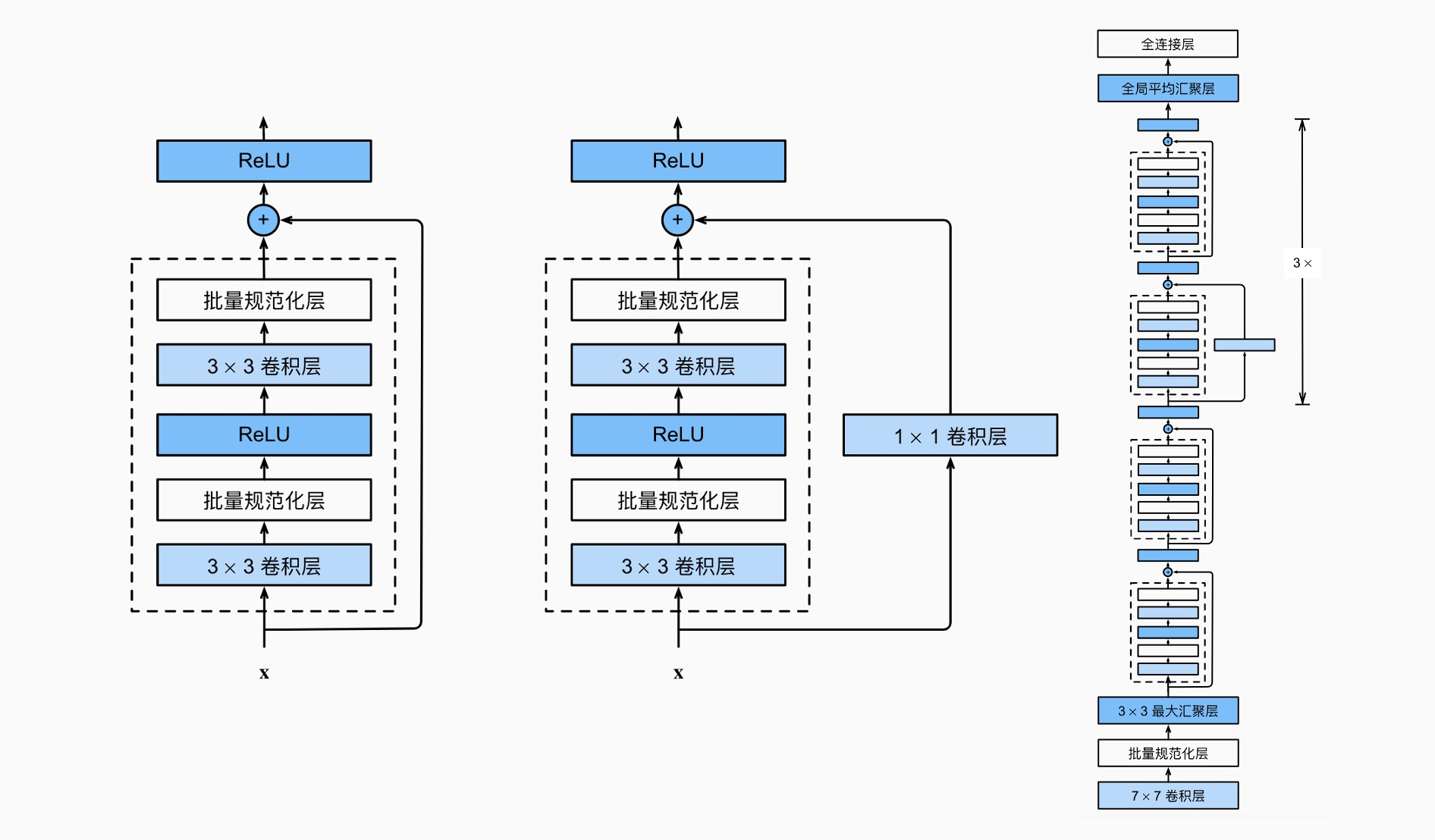### 迁移学习 ¶

• 对于小数据集，仅初始化全连接输出层并重新训练，固定其它层参数，只改变全连接输出层参数
• 对于大数据集，仅初始化全连接输出层和全连接隐藏层，固定其它层参数，重新训练